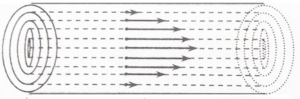Courses

# Mechanical Properties of Fluids: Viscosity Class 11 Notes | EduRev

## Class 11 : Mechanical Properties of Fluids: Viscosity Class 11 Notes | EduRev

The document Mechanical Properties of Fluids: Viscosity Class 11 Notes | EduRev is a part of the Class 11 Course Physics Class 11.
All you need of Class 11 at this link: Class 11

Viscosity

It is the internal resistance to flow possessed by a liquid. The liquids which flow slowly, have high internal resistance. This is because of the strong intermolecular forces. Therefore, these liquids are more viscous and have high viscosity.
The liquids which flow rapidly have a low internal resistance. This is because of the weak intermolecular forces. Hence, they are less viscous or have low viscosity.Laminar Flow

Consider a liquid flowing through a narrow tube. All parts of the liquids do not move through the tube with the same velocity. Imagine the liquid to be made up of a large number of thin cylindrical coaxial layers. The layers which are in contact with the walls of the tube are almost stationary. As we move from the wall towards the centre of the tube, the velocity of the cylindrical layers keeps on increasing till it is maximum at the centre.
This is a laminar flow. It is a type of flow with a regular gradation of velocity in going from one layer to the next. As we move from the centre towards the walls, the velocity of the layers keeps on decreasing. In other words, every layer offers some resistance or friction to the layer immediately below it.
Viscosity is the force of friction which one part of the liquid offers to another part of the liquid. The force of friction f between two layers each having area A sq cm, separated by a distance dx cm, and having a velocity difference of dv cm/sec, is given by:
f ∝ A (dv / dx)
f = η A (dv/dx)
where η  is a constant known as the coefficient of viscosity and dv/dx is called velocity gradient. If dx = 1, A = 1 sq cm; dv = 1 cm/sec, then f = η. Hence the coefficient of viscosity may be defined as the force of friction required to maintain a velocity difference of 1 cm/sec between two parallel layers, 1 cm apart and each having an area of 1 sq cm.

Units of Viscosity

We know that: η = f .dx / A .dv. Hence, η = dynes × cm / cm×cm/sec. Therefore we may write: η = dynes cm-2 sec or the units of viscosity are dynes sec cm-2. This quantity is called 1 Poise.
f = m × a
η = (m × a × dx) / ( A .dv)
Hence, η = g cm-1 s-1
Therefore, η = 1 poise
In S.I. units, η = f .dx / A .dv
=  N × m / ( m2 ×ms-1)
Therefore we may write, η = N m-2 or Pas
1 Poise = 1 g cm-1s-1 = 0.1 kg m-1 s-1

Solved Examples

Q: The space between two large horizontal metal plates 6 cm apart, is filled with a liquid of viscosity 0.8N/m. A thin plate of surface area 0.01m2 is moved parallel to the length of the plate such that the plate is at a distance of 2m from one of the plates and 4cm from the other. If the plate moves with a constant speed of 1ms−1, then: For You
(a) Fluid layer with the maximum velocity lies midway between the plates.
(b) The layer of the fluid, which is in contact with the moving plate, has the maximum velocity.
(c) That layer which is in contact with the moving plate and is on the side of the farther plate is moving with maximum velocity.
(d) Fluid in contact with the moving plate and which is on the side of the nearer plate is moving with maximum velocity.
Ans: (b)
Solution: The two horizontal plates are at rest. Also, the plate in between the two plates, is moving ahead with a constant speed of 1ms−1. The layer closest to this plate will thus move with the maximum velocity.

Offer running on EduRev: Apply code STAYHOME200 to get INR 200 off on our premium plan EduRev Infinity!

## Physics Class 11

73 videos|313 docs|177 tests

,

,

,

,

,

,

,

,

,

,

,

,

,

,

,

,

,

,

,

,

,

;